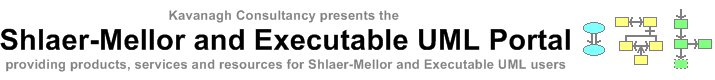# Mathematical Dependence

OOA96 added the concept of mathematical dependence and mathematically dependent attributes to information modelling. Such attributes are annotated with an `(M)` suffix and are known as derived attributes in most other object-oriented methods (including Executable UML). Although the term mathematically dependent is a mouthful and the alternative term derived is much easier to use, it does allow us to distinguish between the analysis and design/implementation concept of derived.

When mathematically dependent attributes are calculated depends on the software architecture. They may be calculated on demand, i.e. whenever their value is accessed. Alternatively, they may be calculated whenever their dependent attributes change and then cached for instant access when needed. However, Recursive Design in OOA10 allows attribute updated mappings to be created in bridges that execute action language code whenever an attribute changes. If such a mapping is made from a particular mathematically dependent attribute then the second approach must be implemented for that attribute.

OOA10 also allows mathematically dependent relationships which are a new type of binary relationship formalized using action language code. Code needs to be provided for navigation in both directions. This code is given an instance of the source participant and returns a set of corresponding instances of the target participant. Using mathematically dependent relationships, analysts can now define composed relationships of the form `R1 = R2 - R3` etc.